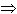SEARCH HOMEMath Central Quandaries & QueriesQuestion from Nigel, a student: I am completely lost...can you help with this: The time, t, to travel a distance, d, at a constant speed, v, can be obtained from the formula: d = vt. If the speed is increased by x, find an expres- sion to represent the decrease in time to travel the same distance. Help!!Hi Nigel.

Let's think of the two different situations: travelling slow and travelling fast:

d = vtt = d/v, so

ts = d/vs and tf = d/vf

since d is the same distance in both cases. What you are asked for is the decrease in time. That's the difference ts - tf :

ts - tf = d/vs - d/vf

Now, you also know that the increase in speed (vf - vs) is x, so can you finish the solution by substituting v for vs and v + x for vf and then simplifying?

Hope this helps,
Stephen La Rocque.Math Central is supported by the University of Regina and The Pacific Institute for the Mathematical Sciences.